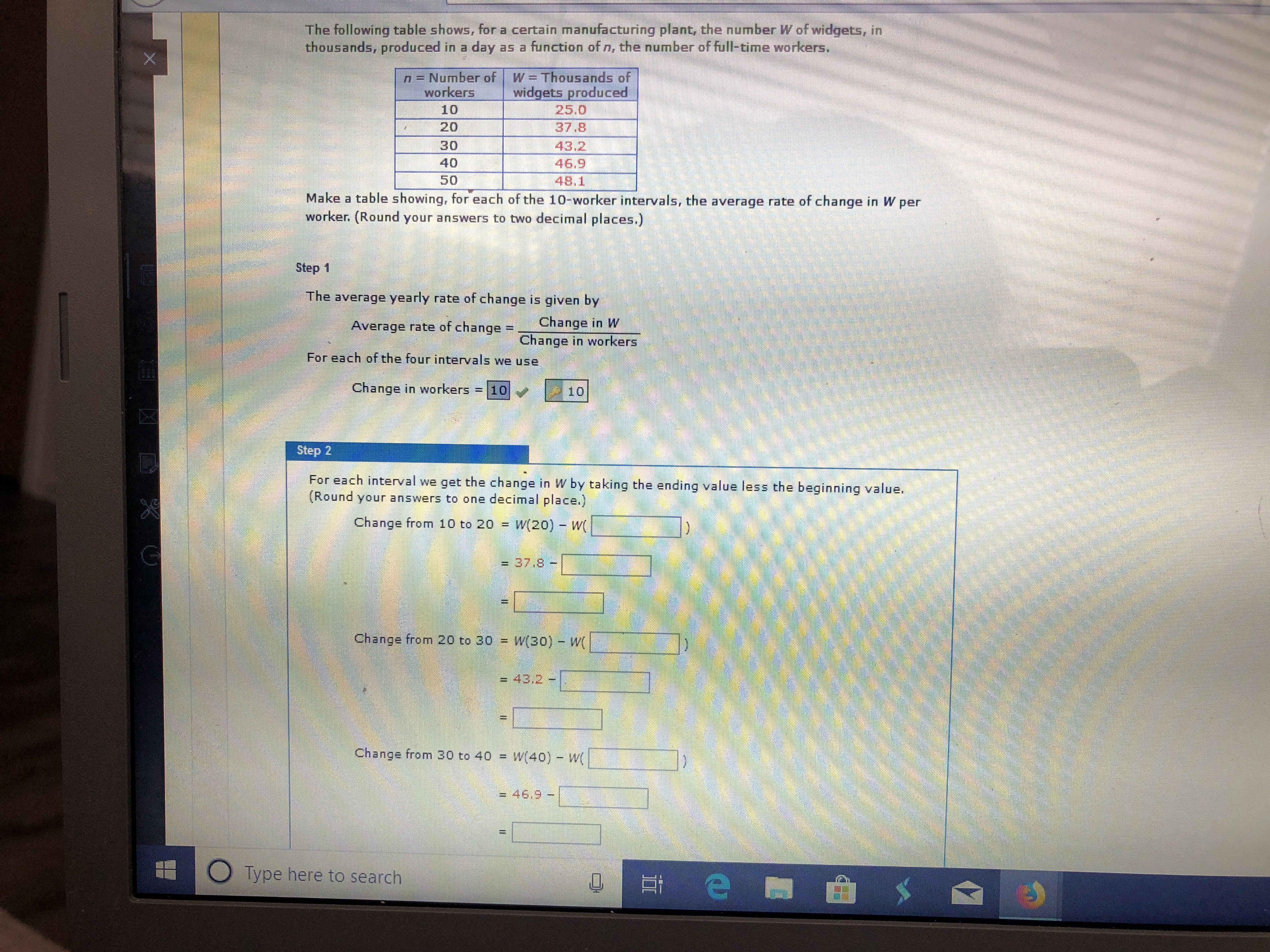The following table shows, for a certain manufacturing plant, the number W of widgets, inthousands, produced in a day as a function of n, the number of full-time workers.XW =Thousands ofwidgets produced25.037.8n = Number ofworkers10203043.246.948.14050Make a table showing, for each of the 10-worker intervals, the average rate of change in W perworker. (Round your answers to two decimal places.)Step 1The average yearly rate of change is given byChange in WChange in workersAverage rate of change =For each of the four intervals we useChange in workers -1010Step 2For each interval we get the change in W by taking the ending value less the beginning value.(Round your answers to one decimal place.)Change from 10 to 20W(20) W(= 37.8-Change from 20 to 30 W(30) -W43.2Change from 30 to 40W40) - W= 46,9-Type here to search

Question

what are the blankshelp_outlineImage TranscriptioncloseThe following table shows, for a certain manufacturing plant, the number W of widgets, in thousands, produced in a day as a function of n, the number of full-time workers. X W =Thousands of widgets produced 25.0 37.8 n = Number of workers 10 20 30 43.2 46.9 48.1 40 50 Make a table showing, for each of the 10-worker intervals, the average rate of change in W per worker. (Round your answers to two decimal places.) Step 1 The average yearly rate of change is given by Change in W Change in workers Average rate of change = For each of the four intervals we use Change in workers -10 10 Step 2 For each interval we get the change in W by taking the ending value less the beginning value. (Round your answers to one decimal place.) Change from 10 to 20 W(20) W( = 37.8- Change from 20 to 30 W(30) -W 43.2 Change from 30 to 40 W40) - W = 46,9- Type here to search fullscreen
Step 1

Change from 10 to 20:

Step 2

Change from 20...

Want to see the full answer?

See Solution

Want to see this answer and more?

Our solutions are written by experts, many with advanced degrees, and available 24/7

See Solution
Tagged in

Algebra